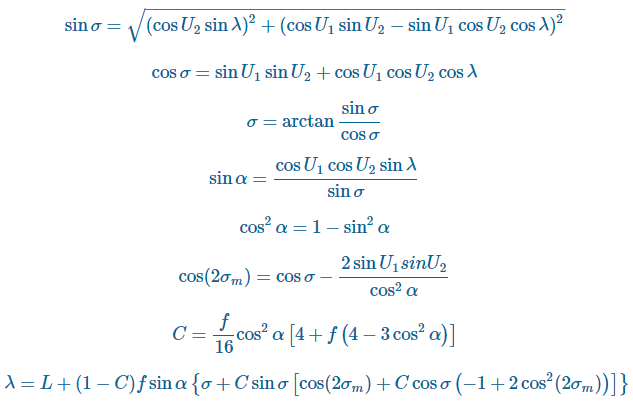# Distance on an ellipsoid: Vincenty's Formulae

11360
0
10-10-2017 10:02 PM
Labels (1)Regular Contributor
2 0 11.4K

Vincenty's Formulae are two methods named after Thaddeus Vincenty a Polish-American geodesist who lived during the 20th Century. During his career, he devised his formulae as well as contributing to major projects such as the NAD 83 where he introduced three-dimensional Earth-centered coordinates. Vincenty's formulae were published in 1975 and it can be downloaded and read here.

The formulae were developed for calculating geodesic distances between a pair of lati­tude/longi­tude points on an ellipsoidal model of the Earth (an oblate sphere). This ellipsoidal geometry is the next step, and perhaps, more appropriate geometry to use when modeling the Earth from working with a sphere using the Haversine Formula see https://community.esri.com/groups/coordinate-reference-systems/blog/2017/10/05/haversine-formula?sr=.... Unlike the Haversine method for calculating distance on a sphere, these formulae are an iterative method and assume the Earth is an ellipsoid.However, even though Vincenty's formulae are quoted as being accurate to within 0.5 mm distance or 0.000015″ of bearing; the Haversine formulas are accurate to approximately 0.3%, which maybe be good enough for your project. Further consideration should be given to the datum being used, for example, WGS-84 is defined to be accurate to ±1m, perhaps negating the 0.5mm distance accuracy quoted for these formulae!1. The Direct Method computes the location of a point that is a given distance and azimuth from another point
2. The Inverse Method computes the geographical distance and azimuth between two given points.

As mentioned above the formulae are iterative processes meaning that a sequence of equations is calculated where the output is reentered into the same sequence of equations. The aim is to minimise the output value after a set number of iterations.

For example, the Inverse Method outputs λ after assigning several constants including the length of the semi-major axis, length of the semi-minor axis, flattening, latitude coordinates, reduced latitudes, etc. The aim of the method is to minimise the value of the output λ (i.e. when the results converge to a desired degree of accuracy):When the difference between the current value of λ and the value of λ from the previous iteration is less than the convergence tolerance then the final stage of the Inverse Method can be executed:It is worth noting that this iterative solution to the inverse problem fails to converge or converges slowly for nearly antipodal points. This issue was discussed and alternative methods proposed in future papers by Vincenty (e.g. Vincenty 1975b).

The code for these formulae is available in Python from the PyGeodesy GitHub project or from Nathanrooy.github.io. Using PyGeodesy, the user can implement the Vincenty methods and change the ellipsoid for example in the below (taken from PyGeodesy GitHub project):

``#from pygeodesy.ellipsoidalVincenty import LatLonNewport_RI = LatLon(41.49008, -71.312796)Cleveland_OH = LatLon(41.499498, -81.695391)Newport_RI.distanceTo(Cleveland_OH)866455.4329158525 # meter#Change the ellipsoid model used by the Vincenty formulae as follows:from pygeodesy import Datumsfrom pygeodesy.ellipsoidalVincenty import LatLonp = LatLon(0, 0, datum=Datums.OSGB36)#or by converting to anothor datum:p = p.convertDatum(Datums.OSGB36)‍‍‍‍‍‍‍‍‍‍‍‍‍‍‍‍‍‍‍‍‍‍‍‍‍‍‍‍‍‍‍‍‍‍‍‍‍‍‍‍‍‍‍‍‍‍‍‍‍‍‍‍‍‍‍‍‍‍‍‍‍‍‍‍‍‍‍‍‍‍‍‍‍‍‍‍‍‍‍‍‍‍‍‍‍‍‍‍‍‍‍‍‍‍‍‍``

I would also recommend having a look at the code samples written by Dan Patterson‌ on his Github Numpy Samples page.